There are all sorts of resonances around us, in the world, in our culture, and in our technology. A tidal resonance causes the 55 foot tides in the Bay of Fundy. Mechanical and acoustical resonances and their control are at the center of practically every musical instrument that ever existed. Even our voices and speech are based on controlling the resonances in our throat and mouth. Technology is also a heavy user of resonance. All clocks, radios, televisions, and gps navigating systems use electronic resonators at their very core. Doctors use magnetic resonance imaging or MRI to sense the resonances in atomic nuclei to map the insides of their patients. In spite of the great diversity of resonators, they all share many common properties. In this blog, we will delve into their various aspects. It is hoped that this will serve both the students and professionals who would like to understand more about resonators. I hope all will enjoy the animations.

Origins of Newton's laws of motion

History of mechanical clocks with animations
Understanding a mechanical clock with animations
includes pendulum, balance wheel, and quartz clocks

## Sunday, May 1, 2016

### Spherical harmonics

 All postings by author previous: Standing waves and rotating waves in three dimensional cylindrical resonators up: Contents next: The Lagrangian approach to simple waves - several common waves that lack momentum
 This posting includes flash animations showing the physics discussed. Most computers have a flash player already installed, but if yours does not, download the free Adobe flash player here. Table of 9 flash animations of animated spherical harmonic modes. Table of 9 flash animations of rotating spherical harmonic modes. Flash animation of tilted axis rotating spherical harmonic mode. Flash animation of spherical harmonic radiation mode. Flash animation of rotating (spiraling) spherical harmonic radiation mode.

Spherical harmonics

Keywords: spherical harmonics, tilted spherical harmonics, rotating waves, standing waves, Flash animation, technical illustration, separation of variables

1. Introduction to spherical harmonics and the Helmholtz equation
Spherical harmonics represent the angular part of a solution of the Hemholtz equation in cases of spherical symmetry. Because the Helmholtz equation governs most simple waves, spherical harmonics can be applied to various cases of spherical symmetry involving waves such as spherical acoustic resonators and quantum mechanical electron waves around an atom. Spherical harmonics are ever present in waves confined to spherical geometry, similar to the common occurence of sinusoids in linear waves.
• A standard approach of solving the Hemholtz equation (∇2ψ = −k2ψ) and related equations is to assume a product solution of the form:

Ψ(r,φ,θ,t) =  R(r) Φ(φ) Θ(θT(t)       ,          (1)

that is, the complicated function Ψ of four variables is assumed to be a product of four functions, each of only one variable.

• Using a process called separation of variables we end up with four differential equations, one for each of the four variables. Each of these equations is a function of only one variable and normally yields nice solutions. A detailed, step by step accounting of the separation of variables process is given in Section 2 below. In the current section we will focus on the outcome of the process.
• For much of this posting we will not be interested in the function R(r), but will instead focus on the groupings of the purely angular part, Φ(φ) Θ(θ), and also on the angular part plus the time part, Φ(φ) Θ(θT(t). In the next section we show an example of using spherical harmonics to calculate acoustic modes in a spherical ball resonator. In that discussion we explain one example of R(r).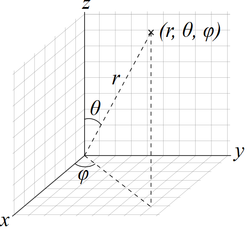Fig. 1. Cartesian and spherical coordinate system used in this discussion [wikipedia].
• #### Spherical harmonics

The purely angular part of the Helmholtz equation i.e. Φ(φ) Θ(θ), has an array of solutions called spherical harmonics which we will examine below.

• #### φ dependence

The φ part of the spherical harmonics has sinusoidal solutions of such as:

Φ(φ) = cos        ,          (2)

where m is an integer. If the situation warrants it, the cosine function can be replaced by the sine function. Solutions also can include linear combinations of the cosine and sine forms. Alternatively we can use complex representation of the sine and cosine functions, i.e. Φ(φ) = eimφ.

• #### θ dependence - Legendre polynomials

The θ part of spherical harmonics has the form:

Θ(θ) = Pm(cos θ)       ,          (3)

where Pm(cos θ) is an associated Legendre polynomial written as a function of cosθ and not of θ itself. Note that m in the above equation is not a superscript, i.e. not representing a power to raise P to, but instead is an index denoting which Legendre polynomial we are addressing. Both and m are integer indices.

• We will not dwell much on associated Legendre polynomials except to note the rules of their indices and m.

• can take on values of 0 to any postive integer (to infinity if desired, although we normally deal with the smaller integers).

• For a particular value of m can take on positive and negative integers from − to 0 to +. For example, if were 4, then m could be any of −4, −3, −2, −1, 0, 1, 2, 3, or 4.

• Each allowed combination of and |m| (absolute value of index m) represents a distinct, mathematically independent solution to the differential equation governing Θ(θ). Below in Table 1 we see some of the associated Legendre polynomials.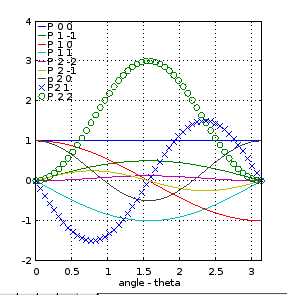Fig. 2. Graphs of the various associated Legendre polynomials shown in Table 1 plotted versus x, for example Pℓm(x) is plotted versus x. Fig. 3. The same Legendre polynomials as in Fig. 2 but now the independent variable in each polynomial is replaced by cosθ and then plotted versus θ. Now Pℓm(cosθ) is plotted versus θ.

• #### θ and φ dependence together - spherical harmonics

As mentioned above, the combination of Θ(θ) and Φ(φ) together is known as spherical harmonics. In the real form they are given by:

Ym(θ,φ) = Km  Pm(cos θ) cos       ,          (4)

where we are required to use the same index m in both the cos  and the Pm(cos θ) parts of (4). Both integer indices and m are bound by the rules discussed above. The Km  are "normalization" constants usually chosen so that the integral of Ym squared over the whole sphere ( 0 ≤ θ ≤ π  and −π < φ ≤ +π ) equals 1.0  [ref].

The first few spherical harmonics are shown in Table 2a below in the real representation. The complex form is shown in Table 2b. In the complex form the cos  in Equation 4 has been replaced by eimφ.

• More on the real representation can be found here. A table of the complex representation is here.
• The coefficients in Table 2a are as appropriate for the real representation of spherical harmonics. Complex spherical harmonics commonly have an extra factor of 1/√2 in the coefficients. This extra factor arises from the complex cases being normalized by requiring that each spherical harmonic times its complex conjugate when integrated over the whole sphere ( 0 ≤ θ ≤ π  and −π < φ ≤ +π ) equals one.
• Also shown are the Cartesian forms of the spherical harmonics.
• We also show the rotating wave representations which are discussed after the table.
• While the two associated Legendre polynomials for a given with indices −m and +m are not mathematically independent, in the case of spherical harmonics these two solutions are mathematically independent due to the addition of sin  and cos . See Tables 2a and 2b for examples of this.

Table 2a. Spherical Harmonics - real form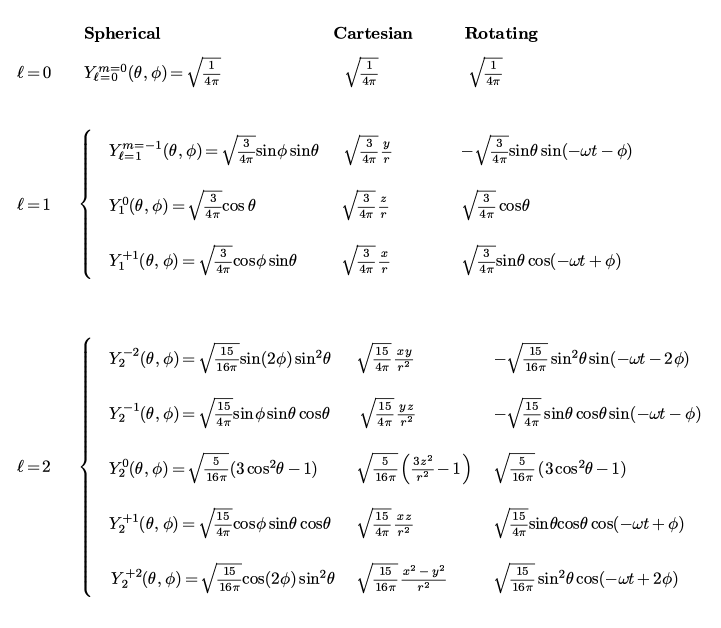Table 2b. Spherical Harmonics - complex form• The time dependent differential equation for T(t) in Equation (1) has sinusoidal solutions such as:

T(t) = cos ωt      ,          (5)

for the real form, where ω is the angular frequency of the solution. So for the complete real solution including θ, φ and t dependence we simply multiply the above spherical harmonics of the first two columns by a sinusoid of ωt such as by cosωt.

• Another option is to use the complex form of the time dependent sinusoid T(t) = e−iωt multiplying a real spherical harmonic which yields a complex solution. On the other hand if we try to simply multiply the complex form of a spherical harmonic by e−iωt we end up with the exponential form of ei(−ωt−mφ) which is the rotating wave form. To get the complex standing wave form while avoiding the problems with multiplying two phasors together, we need to convert one of phasors to the real form first before multiplication. This can be accomplished by using the real spherical harmonic times the e−iωt factor.

• For real rotating wave modes, we combine Φ(φ) with T(t) in the form:

[Φ(φ)T(t)]rotating wave = cos(−ωt + mφ)       ,          (6)

where we are free to substitute sin for the cos function or use the complex representation ei(−ωt + ) as we discussed in reference to Equations (2) and (5). The cos(−ωt + mφ) variety is shown in the last column of Table. 2 above. We shall discuss the rotating wave modes more after the introduction of animations.

• We do not discuss the radial dependence because different applications of spherical harmonics have different radial solutions. In fact some uses of spherical harmonics involve the surface of a sphere in which a radial solution does not have meaning. The angular and time parts of the solution are the same for many applications.

• Below we show three tables of illustrations of various low order sperical modes:
• Pure spherical harmonics (no time dependence)
• Animated standing wave modes using spherical harmonics and sinusoidal time dependence
• Animated rotating wave modes

Table 3. Illustrations of standing wave spherical harmonics - These are of the pure spherical harmonic without added time dependence. A flash animated version of these with added time dependence is shown below in Table 4.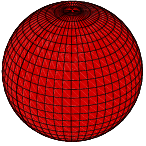= 0, m = 0
Note that the  = 0, m = 0 mode has the same value in all directions. See Fig. 4 below to understand the coordinate system used in these illustrations.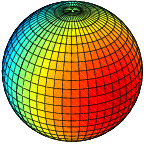= 1, m = −1= 1, m = 0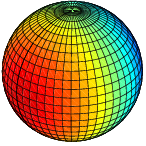= 1, m = +1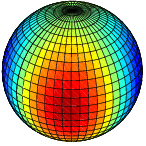= 2, m = −2= 2, m = −1= 2, m = 0= 2, m = +1= 2, m = +2Fig. 4. Cartesian and spherical coordinate system used in Tables 3, 4 and 5 as mapped on a surface of a sphere. We show the φ and θ coordinates of point P.
Note that the higher the absolute value of m the more oscillations the spherical harmonic values go through as φ varies around the circle. For a given m value, larger 's mean more oscillations as θ varies. This table is similar to that found in this reference (scroll down for the illustrations).
##### Relating twin modes to rotating wave modes

Note also that for a given value and |m| > 0 we can consider the modes in pairs. For example as shown in Table 3 above, for  = 2, the m = +2 spherical harmonic is almost the same as the  = 2, m = −2 spherical harmonic. The only difference is that the m = −2 spherical harmonic has been rotated in the φ direction such that its maxima (antinodes) are located where the the other spherical harmonic has its minima (nodes), i.e. rotated by an angle of  φrotatedπ/2m . Because the spherical harmonics can be paired up +m with −m both being practically the same, many references only show the spherical harmonics with positive m values. This is the case with the reference given above. At the same time the two twin modes in a pair are distinctly mathematically independent (linearly independent).ℓ = 2,   m = +2 ℓ = 2,   m = −2 Fig. 5. Top view of a pair of spherical harmonics both having the same ℓ and same magnitude of m but with different signs of m. This drawing further illustrates that the two spherical harmonics in such a pair are the same except that one is rotated in the φ direction by an angle of  π/2m .

A rotating wave mode is simply a sum or difference of two twin standing waves with an added π/2 temporal phase shift between the twin modes. The math behind this was explained in earlier postings: link1, link2. At its core is the trig manipulation of the φ dependence of a spherical harmonic and the time dependence: cos cosωt + sin sinωt = cos(mφ−ωt).

There are many online references that show spherical harmonics as three dimensional objects. These show the values at various angles as colored projections. While these may be appropriate for the use of spherical harmonics when combined with a radial dependence, such as for solving for the electron wave function in a hydrogen atom, they are misleading in that they imply that spherical harmonics by themselves involve a radial component. We believe coloring the surface of a sphere correctly implies the purely angular nature of spherical harmonics.

Table 4. Animations of time varying standing wave spherical harmonics

= 0, m = 0
These animations show the time dependence added to the spherical harmonics as per Equation (5) above.
Mouse over each image to see the animation, mouse off to suspend it and click on it to restart it.
Viewing these animations requires a free adobe flash viewer on your computer. Many computers already have this viewer installed.

= 1, m = −1

= 1, m = 0

= 1, m = +1
Note that the three modes are the same except they are oriented in three different directions, along the x, y and z axes.

= 2, m = −2

= 2, m = −1

= 2, m = 0

= 2, m = +1

= 2, m = +2

Table 5. Animations of time varying rotating wave spherical harmonics

= 0, m = 0
These animations show the time dependence added to the spherical harmonics as per Equation (6) above.
Mouse over each image to see the animation, mouse off to suspend it and click on it to restart it.
Viewing these animations requires a free adobe flash viewer on your computer. Many computers already have this viewer installed.

= 1, m = −1

= 1, m = 0

= 1, m = +1

= 2, m = −2

= 2, m = −1

= 2, m = 0

= 2, m = +1

= 2, m = +2

### 2. An acoustic spherical resonator - details of separation of variables

The wave equation for the acoustic pressure p (the oscillating component of the pressure due to an acoustic resonance) is:

where c is the speed of propagation of a plane wave front in the medium under consideration. The Laplacian (i.e. the ∇2) in spherical coordinates makes this:

Following the separation of variables method, we assume a product solution:

where R(r) is only a function of r,  Θ(θ) is only a function of θ,  Φ(φ) is only a function of φ  and T(t) is only a function of t.

#### Extracting the time dependence:

When we substitute (12) into (11) and divide by R(r)⋅Θ(θ)⋅Φ(φ)⋅T(t) we get:

We see that the function on the right depends only on time t while the function on the left depends on the other variables and not on time. This requires that both sides equal a constant, a separation constant which, in hindsight, is negative the wavenumber squared, i.e. −κ2. So we have two equations now:

and

The second equation has sinusoidal solutions of ωt such as:

where ω =  is the angular frequency.

#### Extracting the φ dependence:

Equation (14) can be rearranged as:

which again can be separated with a separation constant of −m2 and yields the equations:

Equation (19) has sinusoidal solutions of such as:

where B is a constant (the amplitude). We discuss this solution above.

#### Extracting the θ dependence:

Equation (18) can be rearranged as:

with the left side only being a function of r and the right side only a function of θ. We use the separation constant  ( + 1)  to yield the following two equations:

Equation (23) can be rearranged as:

If we let  x = cosθ ,  so that  sin2θ = 1 - cos2θ = 1 − x2  and  dx = −sinθ   then our equation becomes:

which yields:

which is the same form as the general Legendre equation which has solutions of associated Legendre polynomials Θ(x) = Pm(x) or written as:

as noted above.

#### Extracting the radial or r dependence - spherical Bessel functions:

Turning our attention to the radial dependence, Eqn. (22) can be rewritten as:

According to Wolfram, the "transformed" version of Bessel's equation is:⇑ Fig. 6. First three spherical Bessel functions, jn(x) for n = 0, 1, 2. Wikipedia.⇑ Fig. 7. First three spherical Neumann functions, yn(x) for n = 0, 1, 2.

and has solutions of:

where q is defined as:

We can discard the second term in (30) because of Y's singularity at the origin which is included in our spherical resonator. Also if we compare (28) and (29) we see that in our case: p = ½,  a = κr = 1,  β = i((l + )),  and q = √(¼ + ( + 1)) = ½√((2 + 1)2) =  + ½.

##### Spherical Bessel functions:

This makes our solution for R(r):

where D is a constant and the j(κr) are "spherical Bessel functions" defined as:

As (33) suggests, spherical Bessel functions can be considered as a special subclass of regular Bessel functions having half integer orders. Alternatively, they can be related to sinusoids in a much simpler fashion than normal integer-order Bessel functions can be. The relations between the first few spherical Bessel functions and sinusoids are:

#### Putting the whole solution together:

Back to the larger problem of the solutions for acoustical waves inside a spherical enclosure, we assemble the parts above to give:

where ω/κ = c and c is the speed of sound. Eqn. (35) specifies a set of solutions with being any positive integer or 0 and m being any positive or negative integer or 0 in the range of  − ≤ m ≤ + .   The wavenumber κ can be adjusted to fit the boundary condition at the interface with the spherical container which will affect the resonant frequency ω via the equation  ω/κ = c.  The amplitude E can be adjusted to fit the intensity of the captured waves. A general solution will involve sums of these solutions. A general solution may also substitute other sinusoids in for the cos and cosωt.

#### Resonant frequencies of a spherical resonator

To calculate the frequencies of the resonant modes of our acoustic spherical resonator we need to adjust the radial wavenumber κ so that the boundary conditions are met. This means that if we have Dirichlet boundaries we need the R(r) factor in (35) to be zero at the resonator's radius. Since R(r) is proportional to j(κr) this means j(κa) = 0  at r = a where a is the radius of the resonator. This means κa = uℓ,n or:

κ = uℓ,n /a       ,          (36)

where uℓ,n is the nth root of j .

Similarly for Neumann boundaries, the derivative of j(κr) with respect to r must be zero at r = a. Thus:

κ = u'ℓ,n /a       ,          (37)

where u'ℓ,n is the nth root of the derivative of  j .

We might note that in Equations (28) through (37) nowhere is the index m refered to. This means that the resonant frequencies do not depend on m and only depend on and n. So when we examine Tables 4 and 5 above, we should remember that all the modes with the same will have the same resonant frequencies as long as the n index is also the same. Thus, except for the top most mode of the chart ( = 0), all the modes are degenerate with other modes. Since there are 2 + 1 modes for a given , we should expect a 2 + 1 degeneracy. This can be broken if we introduce a perturbation to make the resonator a little asymmertric.

3. Special functions in physics

During the last few centuries mathematicians and physicists created many special functions (2nd ref) such as the above mentioned spherical Bessel functions. These are documented in classic references such as Abramowitz, Milton; Stegun, Irene Ann, eds. (1983) [June 1964]. Handbook of Mathematical Functions with Formulas, Graphs, and Mathematical Tables and many other places. These are mathematical functions, each of which is the solution to a special differential equation governing some physical process in nature. The best known of these are trigometric functions (sine, cosine, etc) and exponentials (ex).

Each of these functions have all sorts of theory and mathematical relationships, generated by mathematicians and physicists down through the years. The above reference by Abramowitz and Stegun lists many of these relationships. The trick for casual users is not to get too caught up in the heavy overhead of these special functions, but just to consider each a solution to an equation that has been provided to you as a gift by reseachers in the past.

My philosophy is to absorb as much or as little information as required on the special function under consideration to provide a solution to a particular physics problem at hand and then move on.

4. A symmetry dilemma - axis of rotation in a spherically symmetric resonator
 Fig. 8. Flash animation of a rotating wave mode with the axis of rotation different than the z axis. This solution is composed of a sum of modes as prescribed by (40) below. It shows the ℓ = 2, m = 1 mode tipped away from the spherical coordinate system axis shown by the grid. This animation uses the color black for near zero areas of the sphere instead of yellow/green/turquoise used above. Mouse over the image to activate it, mouse off to suspend it, and click on it to restart it.

Above we have been considering the solutions to waves inside a spherical resonator. Such a resonator is totally spherically symmetric with no distinguishing axis or direction. The confusion is: why do most of the solutions have a distinct axis and are not spherically symmetric?

•The universe is full of examples of just this. Take for example our solar system. It is basically defined by the central gravitational force of the sun. This force is spherically symmetric having no preferred axis or direction. At the same time the orbits of the planets do pretty much lie in a plane with a clear orbital axis. This plane and axis is caused by the net angular momentum that the masses that formed the planets brought with them during the formation process. So the axis was defined by an initial condition of the system and not by the central force. The same can be said about galaxies. So concerning our waves inside a spherical resonator, we might expect that the initial condition, or in this case the position of the driving oscillation, might define an axis, since the container itself does not.

• In wave media that allow traveling waves to possess momentum, rotating waves will possess angular momentum. Just as in the case of the solar system, the angular momentum imparted to the wave system will define an axis. More generally, in order to even have rotating waves, there must be an axis of rotation which means we are back to an axis for the solutions.

Another analogy might be in modeling the motion of a basketball (or any sort of ball). The ball is spherically symmetric. Simple Newtonian mechanics will dictate its trajectory through space but we all know that a ball can have spin and this means a spin axis. So even though it is spherically symmetric, a complete description of its motion must include its spin and a spin axis. In a more general sense, spin is an integral part of the motion of three dimensional objects.

##### Sums of modes:
• Another approach is to say that the axis of the solutions is just a mathematical thing, that it is somewhat arbitrary. The nicely written-out solutions happen to be tied to the z axis but we could really choose any axis. By having an axis we allow for spin. The theory of spherical harmonics shows that the set of spherical harmonics form a complete basis for all possible solutions [ref]. So any solution can be alternately written in terms of a sum of solutions written for a different axis. Thus there is a convenient way to mathematically change the axis: in terms of standing waves where the constants Aℓ,m,m'  can be solved for using the orthogonality of spherical harmonics:

integrated over the entire sphere.

• To put time into (40) we have for the wavefunction (ignoring the radial dependence for now):

for standing wave modes where Km' are the constants in (4) above. Also, sin  needs to be substituted for cos  for terms for which m' < 0 as shown in Table 2a above. Equation (41) can be used to calculate the constants Aℓ,m,m' .  The complex form could also be used substituting e−iωt in for cosωt. Do not also substitute the complex sinusoid in for cos because of the problems with multiplying two phasors together unless you want a rotating wave mode.

Rotating wave modes can be written in the real form as:

where ei(−ωt+mφ) could be substituted in for cos(−ωt+) for the complex expression.

• In summary: we solved the wave equation for a spherically symmetric resonator and got a general solution. This being a general solution, it must allow spin states (rotating wave solutions). In nature these spin states can occur with an arbitrary axis orientation. While our solutions are easiest to interpret with the spin axis aligned in the z direction, using (40) through (43) (or just rotation of coordinate system), our set of solutions can accommodate any orientation of the spin axis.

5. Student experiment - vibrational modes of a spherical balloon
Using the setup described in an earlier posting (Fig. 48a) one can excite spherical harmonics on a spherical balloon. Inflate the balloon and suspend it by a string above and a little off center of the loud speaker. Lines drawn on the balloon will help in seeing the motion. Heavy-weight rubber balloons, full but not inflated to the max, seem to work best. An example of a heavy weight spherical balloon.

This balloon experiment will allow students to see the spherical harmonic modes in real life that are mathematically described above and see the succession of modes as one scans through the frequencies. Next we briefly show that the Helmholtz equation governs motion of the surface of a balloon meaning that we should expect spherical harmonic modes in its vibrations.

##### A little math to show the relevance of spherical harmonics to the surface of a balloon:

In this discussion, we assume that most of the wave action is due to the balance between the outward pressure force and inward tensional force of the stretched balloon. We neglect any wave resonance of the air inside the balloon because such resonance would be in the kilohertz range, instead of the Hertz range that we experience.

Similar to the waves on a string case, the inward tensional force is proportional to the curvature of the balloon's surface.

• In the waves on a string case, this force equals T ∇12η where T is the string tension, η(x) is the transverse displacement from equilibrium and ∇12 is the one dimensional Laplacian, i.e. the second derivative with respect to location x.
• In the 2D diaphragm and balloon cases, we expect the unbalanced restoring force to similarly be given by Ts22η where Ts is the surface tension due to the stretched membrane or balloon (in units of Newtons/meter),  ∇22 is the 2D Laplacian and η is the transverse displacement from equilibrium.
• In all three of these cases, Newton's second law gives T ∇2η = ρ ∂2η/∂t2 which is the wave equation where ρ is the density of the string or membrane: mass per length or per area whichever is appropriate. If we are considering a single frequency ω then the last term becomes −ρω2η which means that the equation can be rewritten as ∇2η = −k2η  where k = ωρ/T  = ω/c  is the wavenumber and c is the speed of wave propagation. This last form is the Helmholtz equation, the very same equation that we started this posting on.
• In the case of the balloon, the governing equation is  ∇θ,φ2η = −k2η  where  ∇θ,φ2  represents the θ and φ terms of the spherical Laplacian and η is the radial displacement from equilibrium of the balloon's surface. The solutions to this equation are spherical harmonics as discussed above.

 Figs. 10 and 11. Flash animation and video of spherical radiation pattern for the ℓ = 4, m = 2 mode as prescribed by (50) below. One quadrant has been sliced from the sphere to show the radiation waves propagating outwards to the surface. It is assumed that the waves are either absorbed at the surface or continue to travel outwards unseen past the surface. This animation uses the color black for the near zero areas of the sphere similar to that used in Fig. 8 above. Mouse over the image in Fig. 10 to activate it, mouse off to suspend it, and click on it to restart it. Fig. 11 is started by clicking on the arrow. Because of its complexity the flash animation runs slow on slower computers. The video in Fig. 11 will run faster but is a much larger file to download.

The radiation patterns for waves in three dimensions coming from a small source are described by spherical harmonics. These waves are similar to the two dimensional case previously discussed. In the three dimensional case, for radiation propagating straight away from the source we use the following equation:

Note that we use both j(κr) and y(κr) since the origin is not included in our space (it must be excluded because a radiation field will be infinite there since radiation fields fall off as 1/r). It is the interplay of the two kinds of spherical Bessel functions that mathematically provide for the outward propagation of the waves (similar to the interplay of J(κr) and Y(κr) in two dimensional radiation).

An animation of the  = 4, m = 2 radiation mode is shown in Figs. 10 and 11 above (flash and mp4 video versions). Note that to create particular radiative mode such as as the one shown, the launching "antenna" must be properly shaped for that one mode. Alternately, a phased array with its radiative elements covering the surface of a sphere could also be programmed to a lauch particular radiative mode.